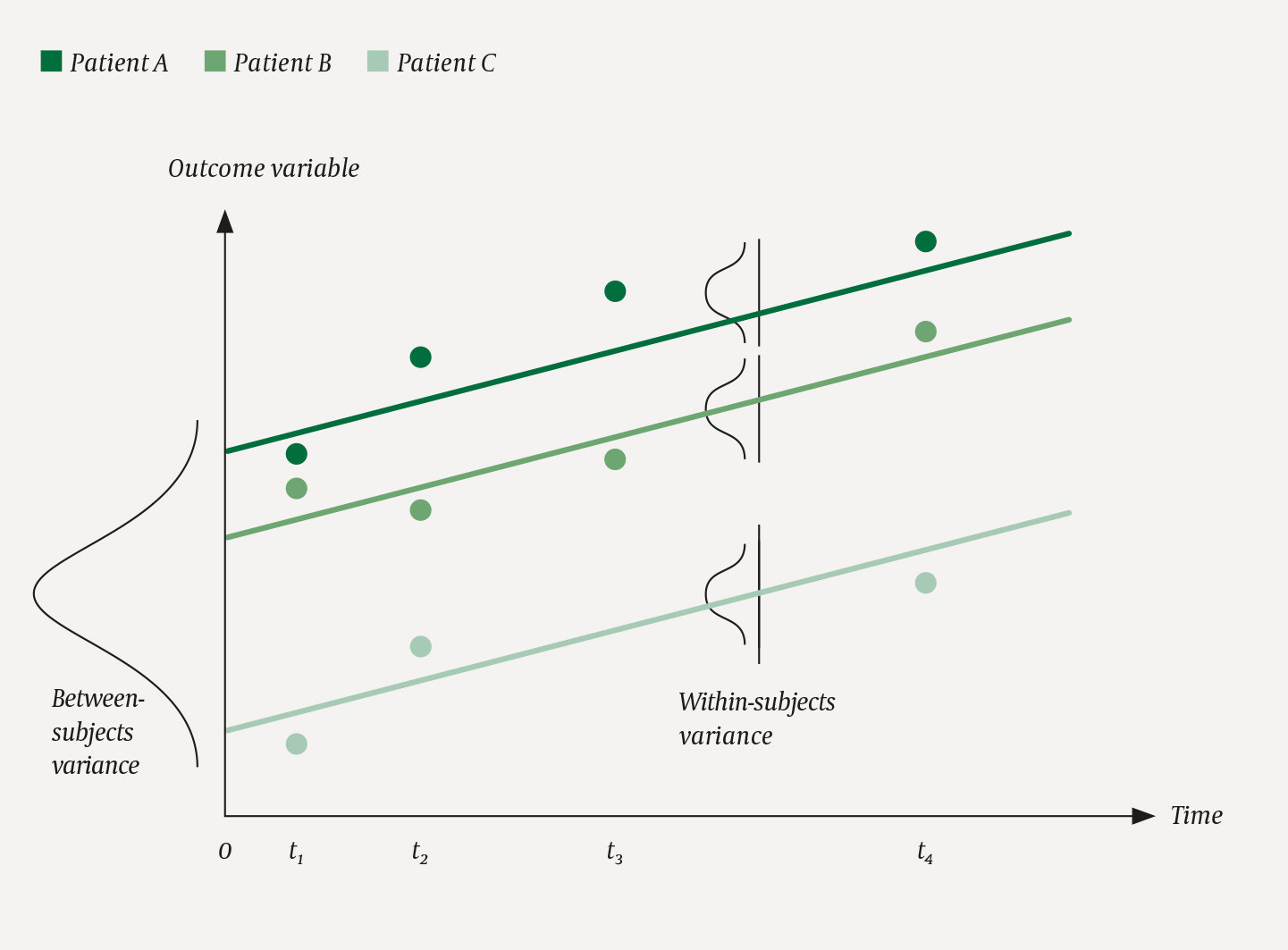# Analysis of longitudinal data

Article

In some studies, the outcome variable is measured several times for the same person, for example in studies where the patients are examined at more than one follow-up time point. In this case, a statistical method for longitudinal data must be used.

Let us begin with an example: in a randomised controlled trial, we compared two courses of treatment for patients with hip fracture (1). The primary outcome variable was mobility, measured by the Short Physical Performance Battery (SPPB) screening test, which produces a variable with scores on a scale from 0 to 12. Patient mobility was measured four times: five days, one month, four months and twelve months after surgery. Figure 1 gives a somewhat simplified presentation of how the course of treatment might have looked for three patients measured at four points in time.Figure 1 Development over time for three fictitious patients, A, B and C, measured at four points in time.
Linear mixed model

## Linear mixed model

Various statistical methods can be used to analyse longitudinal data. A regression model can be a natural starting point. Here, we will focus on a linear mixed model, where we assume that each subject has its own regression line, as shown in Figure 1. The individual measurements vary around the line, with a within-subjects variance. The regression lines also vary between each other. This is quantified with a between-subjects variance. In Figure 1, the regression lines are parallel. This is obviously a simplified model of reality, but in many studies it can fit quite well.

This is referred to as a mixed model, because it contains at least one fixed effect, here the effect of time, i.e. the slope, and at least one random effect, here a random effect of the subject, expressed as the between-subjects variance. If the development over time does not follow a straight line as in Figure 1, we can for example use indicator variables for the points of time t1, t2 etc. with a separate slope for each point of time. This was done in the abovementioned study (1).

One of the advantages of a mixed model is that it includes data from all individuals in the calculations, even those for whom data are missing at one or more time points, such as Patient C in Figure 1. Moreover, the results will be unbiased even if data are only missing at random (2). If individuals with low values at the outset, such as Patient C in Figure 1, have a larger proportion of missing values at later points in time, data are not missing completely at random, but possibly missing at random.

A key use of a mixed model is to study whether the change over time depends on an exposure, for example a treatment. In that case, the interaction term between exposure and time is included to enable investigation of whether the slope differs between the exposure groups.

A linear mixed model can also include multiple independent variables, similar to a standard regression analysis. For example, it may be relevant to include age and sex as confounders in an observational study, or as key predictors of the outcome variable in a randomised controlled trial (3), which was done in the abovementioned study (1). Note, however, that the baseline value of the outcome variable shall normally not be included as an independent variable, but as a dependent variable at baseline. We will return to this in a later article on randomised, controlled trials.

An alternative method

## An alternative method

Previously, a method called Repeated Measures ANOVA was widely used. This method has many disadvantages: the mathematical model is not very transparent, the results are difficult to interpret, only individuals with complete data are included in the analysis, and the results are unbiased only if data are missing completely at random. Today, the Repeated Measures ANOVA method is not recommended (4).

A linear mixed model can generally be recommended for longitudinal data with a continuous outcome variable.

## Recent Articles

Made by Ramsalt Using Ramsalt Media